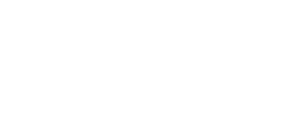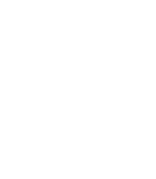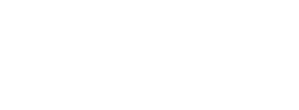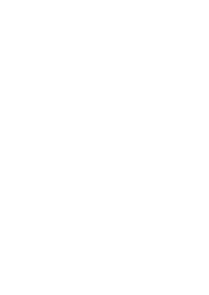# Commercial Arithmetic 2 Questions and Answers - Form 3 Topical Mathematics

## Questions

1. Chepkemoi bought a new washing machine for Kshs.420,000. Its value depreciated over
the next 5years at the following rates; 15%, 13%, 12%, 9% and 7%. For the next 6 years,
the rate of depreciation remained constant at 5% then the rate of depreciation remained at 4%
each. How long did it take for the value of the washing machine to be
1/3 of its original value?
2. The table below shows income tax rates for the year 2006
 Taxable income in shs. Pa Rate of tax in % 1 – 120,000 10 120,001 – 240,000 15 240,001 – 360,000 25 360,001 – 480,000 35 Over 480,000 50
Nafula is married and claims a tax relief of shs.1,120 per month. She stays in a company house For which she pays a nominal rent of shs.1200 per month. She found that in a particular month, her employer deducted shs.4830 as tax. If she is entitled to a maximum insurance policy; relief of shs.600 per month. Calculate her monthly salary. (10mks)
3. The figure below represents two pulley wheels, centres A and B with a rubber band CDEFGHC stretched round them.Radius of wheel centre A = 16cm, AB = 30cm. CD,
GF are tangents to the circles ∠ CAB = 86.3°
1. calculate the length of the belt CD
2. Find the angle ABD
3. Find the length of the belt that would go round the pulleys (CDFGHC)
4. In the figure below, ABCD is a cyclic quadrilateral and BD is a diagonal. EADF is a straight line, ∠CDF = 68o, ∠BDC = 45o and ∠BAE = 98o.Calculate the size of:
1. ∠ABD.
2. CBD
5. A customer deposited Ksh.15,500 in a savings account. Find the accumulated amount after 3½ years if interest was paid at 16% per annum compounded semi-annually
6. A retailer mixes three types of rice, Bismatti costing shs.120 per tin with Pishori costing shs.150 per tin and Ahero rice costing shs.80 per tin in the ratio x : 1 : 2 respectively. If he sells the mixture at shs.137.50 per tin making a profit of 25%. Calculate the value of x.
7. Ashanti is a saleswoman and earns a commission on sales based on the monthly rates shown in the table below:-
 Sales (Kshs) Commission rate % of sales The first 5000 10% The next 3000 15% Sales above 8000 20%
In addition, she earns a basic monthly pay of Kshs.6700. During a certain month, she earned a total salary amounting to Kshs.8368. How much worth of sales did she make?
8. The table below shows the annual income tax rates for a certain year.
 Total income per month in Kshs. Rates in Kshs. Per £ 1-1016410165 – 1974019741 – 2931629317 – 33892388983 and above 2  3  4  5 6 Automatic personal relief shs.1162
Kiptoo earns a monthly salary of Kshs.25000. He is entitled to house and medical allowances of Kshs.12000 and Kshs.3000 respectively
Calculate:
1. His taxable income per month
2. His monthly tax payable
3. His annual tax payable
9. A company employee earns a basic salary of Kshs.25,000 and is also given taxable allowances amounting to Kshs.10,480. Using the table of taxation above:-
1. Calculate the employee’s taxable income
2. If the employee is entitled to a personal tax relief of Kshs.800 per month, determine the net tax
3. If the employee was given 40% increase in his income, calculate the percentage increase in his income tax
10. A certain amount of money was invested at compound interest of 10% compounded every two years for ten years. Given that the investor invested a total of 500,000/= at the end of the ten years, find the amount of money invested to the nearest shillings
11. The cash price of a T.V set is Ksh. 26,000. Linda bought the set on hire purchase terms by paying a deposit of Ksh. 6,000 and the balance by 24 equal monthly installments of Kshs. 1,045.30. Find the compound rate interest per year.
12. What would Kshs. 15000 amount to after 3 years at 16% per annum compounded quartely?
13. Income rates for income earned were charged as follows:
 Income in Kshs. p.m. Rate in Kshs per sh.20 1 - 8400 8,401 - 18,000 18,001 - 30,000 30,001 - 36,000 36,001 - 48,000 48,001 and above 2 3 4 5 6 7
A civil servant earns a monthly salary of Ksh.19,200. His house allowance is Ksh12,000 per month. Other allowces per month are transport Ksh.1300 and medical allowance Ksh.2300.
He is entitled to a family relief of Kshs. 1240 per month.
Determine:
1. His taxable income per month.
2. Net tax.
NHIF                                      shs. 230
Service charge                      Kshs. 100
Loan repayment                    Kshs. 4000
Co-operative shares of           Kshs. 1200
Calculate his net salary per month
14. Use the taxation rates in the table below to answer the questions that follow;-
 Taxable income in K £ p.a Rate % per K£ 1-45004501-75007501 – 1050010501 – 1350013501 – 165000ver 16500 101520253035
The manager of a certain company is entitled to a monthly personal relief of shs.3000 and her tax (PAYE) is kshs.9000 per month she is also deducted NHIF shs.350 per month, WCPS shs.800 per month and cooperative shares shs.1200 per month, calculate
1. The managers total deductions per month
2. Total tax per month
3. The manager’s annual gross salary
4. The manager’s monthly basic salary if her monthly allowance and medical allowances are 10000 and 2000 shillings
15. The table below shows the income tax for a certain year;
 Monthly taxable income (Kshs.) Tax rates (%) 1- 96809681- 1880018801 – 2792027921 – 3704037940 and above 10%15%20%25%30%
In that year, Odero paid a net tax of Kshs.5,512 per month. His total monthly taxable allowances amounted to Kshs.15,220 and he was entitled to a monthly personal relief of kshs.1,162. Every month the following deductions were made;
N.H.I.F                             Kshs.320
Union dues                       Kshs.200
Co-operative shares          Kshs.7,500
1. Calculate Odero’s monthly basic salary in Kshs
2. Calculate his monthly salary
1. A car is worth shs.800,000 when new. During the first year it depreciates by 20% of its value and in the second it deprecates by 5% of its value at the start of the year. During the third, fourth and fifth year, depreciation rate is 10%. How much less will it cost at the end of the fifth year?
2. Find by how much the compound interest will exceed simple interest on shs.3,000 for two years at 15% per year
16. The table below shows the income tax rates:

Income per month (K
£        Rate in Kshs per £
1 - 325                                                2
326 – 975                                            3
976 - 1300                                           5
1301 – 1625                                         6
Over 1625                                            7.50
Mr. Misoi is a public servant who lives in a government house and pays a nominal rent of Kshs.1,220 per month. He earns a basic salary of Kshs. 24,800 and a house allowance of Kshs.12,000 per month. He is entitled to a monthly relief of kshs.1620.
1. Calculate his monthly;
1. Taxable income in K£
2. Tax payable without relief
3. Tax after relief
2. Apart from the income tax. The following monthly deductions are made from his earnings
-HELB loan repayment Kshs.2400
- Health insurance fund Kshs.1200
- 2% of Basic salary union fee
Calculate:-
1. the total monthly deduction made on Mr. Misoi’s income
2. Mr. Misoi’s net income per month
17. Joseph bought a camera on hire purchase (H.P) term by paying a deposit of shs.7200 and cleared the balance in 24 equal monthly installments each of 1250.
1. find the hire purchase price of the camera
2. the hire purchase price of the camera is 24% higher than the cash price. Find the cash price of the camera
3. Kangara took a loan from a financial institution and bought the camera with cash. He repaid the loan at 18% p.a compound interest at the end of the two years. Find the total interest paid by Kangara.
18. Income tax for all the income earned was charged at the rates shown.
 Total Income p.a (K.£) Rate in sh per K£ 1 – 1980 1981 – 39603961 – 64406441 – 79207921 – 9900Excess of 9900 2  3  5  7  9 10
1. Wanyonyi earned a salary of Kshs.10,500 per month. In addition he was given a house allowance of Kshs. 6500 per month. He got tax relief of Kshs. 300 per month.
Find ;
1. His taxable income p.a
2. Income tax he pays per month.
2. A part from income tax the following deductions are made per month. NHIF of Kshs

1. After 1st year = (95/100 x 4200000)
= Shs.357,000
After 2nd year = ( 87/100 x 357000)
= sh310590
After 3rd year = (88/100 x 310590
= shs.273319.20
After 4th year = ( 91/100 x 273319.20)
=shs.248720.50
After 5th year = (248720.50 x 93/100)
The next 6 years = shs. 231310
A = 231310 (1- 0.05)6 = 170034.10
Then 140000 = 170034.10 (1-0.04)n
(0.96)n = 140000/170034.10 = 0.8234
n = log 0.8234/
log 0.96
= 0.0844/0.01773 = 4.76yrs
Total no. of years = 5 + 6 + 4.76yrs = 15.76years
2. Gross tax = 4830 + 1120 + 600 = sh 6550 per month
Annual gross tax = 6550 X 12= 78,600
10 X 120,000 = sh.12,000
100
15 X 120,000 = sh.18,000
100
25 X 120,000 = sh. 30,000
100
Re. tax = 78600 – (12000 + 18000+30000)
=78600 – 60,000 = 18,6000
35 X x =18,600
100
0.35x = 18,600
x = sh 53142.86
Taxable income p.a = 36,000+53142.86 =sh.412142.86
Monthly salary = 413142.86/12 + 12,000
= 34428.57 + 1200 = Sh 35628.57
3.
1. Sin 86.3° = XB/AB
Sin 86.3°= XB/30
XB = 30sin86.3°
XB = CD = 29.93746855 cm
2. ∠ ABX = 90° - 86.3°
= 3.7°
∴∠ ABD = 3.7° + 90°
= 93.7°
3. ∠ DBF obtuse = 360° - 187.4°
= 172.6°
Arc DEF = ø/360 πD or ø/360 x2πr
But cos 86.3° = AX/AB
Cos 86.3° = AX/30
AX = 1.935969248 cm
DB = 16 – 1.935969248 = 14.06403075 cm
Arc DEF = 172.6°/360° 22 /7 x 14.06403075
= 106807.8751
2520
= 42.38407742 cm
Arc CGH
reflex CAG = 360° - (2x 86.3°)
= 187.4°
∴Arc CGH =187.4°/ 360° x2 x22 /7 x 16
= 131,929.6
2520
= 52.35301587 cm
Total length of belt to go round the belt
= CD + DEF + GF + CHG
= 29.93746855 + 42.38407742 + 29.93746855 + 52.35301587
= 154.6120304 cm4. ABD = 310
CBD = 370
5.  A = 15,500(1 + 8/100)7
= Ksh.25,707
6.7. Commission earned Kshs. (8368 – 6700) = Kshs. 1668/=
let sales in 3rd bracket be y
10/100 x 5000) + (15/100 x 3000) + (20/100 x y) = 1668
500 + 450 + 0.2y = 1668
0.2y = 1668 – 950 = 718
y =718/0.2 = 35%
Total sales = (8000 + 3590)
= shs.11590
8.
1. Taxable income = (25000 + 12000 + 3000)= 40000
2. Income tax
10164 x 2/20 = Shs.1016.40
10164 x 3/20 = Shs.1524.60
10164 x 4/20 = Shs.2032.80
Remaining :
9508 x 5/20 = Shs.2377
Total tax payable p.m = 6950.8 – 1162 = Shs.5788.80
3. Annual tax payable = 5788.80 x 12 = Shs.69465.60
9.
1. taxable income = Kshs. 25000 + Kshs.10480
= Kshs. 35480
2. tax charged:
1st 4350 = 4350 x 2/20 = 683.25
2nd 4555 = 4555 x 3/20 – 683.25
3rd 4555 = 4555 x 4/20 – 911
4th 4555 = 4555 x 5/20 - 1138.75
Rem. 17465 = 17645 x 6/20 – 5239
Total tax = 8407.5 - 800.00 = 7607.50
3. 40/100 x 35480 – 14.192 = 49672
New income = 35480 + 14192 = 49672
Remainder = 49672 – 18015 = 31657
Tax charged = 31657 x 6/20 =12665.1
Total tax = 12665.1
% increase in income ax = 4257.6 x 100
7607.5 = 55.97%
10. A = P(HR/100)n
500000 = P( 1+ 20/100)5
500,000 = (120/100)5
500,000 = P
(1.2)5
P = Shs.200,938.786 ≈shs. 200,939
11. Principal = 26, 000 - 6,000 = 20,000
Total H.P instalments = 1045.3 x 24= 25087.20
25087.20 = 20,000 [1/100 + r]2
1.254 = [1+ r/100]2
1.120 = 1/100 + r
r/100 = 0.12 or 12%
12. No. of periods =12
r = 4% per period
A = 1.0412 x 15000
= 24015.5
13.
1. Taxable income = 19200 + 12000 + 1300 + 2300 = 34800
2. Net tax
8400 x 2/20 = 840
9600 x 3/20 = 1440
12000 x 4/20 = 2400
4800 x 5/20 = 1200/5800
Net tax = 5800 – 1240
= 4560
3. Net salary = 34800 – (4560 + 5530)
= 24710
14.
1. 9000 + 350 + 800 + 1200 = 11350
2. 9000 + 3000 = 12000
3. Total taxes = 12000 x 12
= shs.144000p.a
Taxes
450 x 2 = shs.9000
3000 x 3 = shs.9000
3000 x 4 = shs.12000
3000 x 5 = shs.15000
3000 x 6 = shs.18000
Shs.63,000
144000 – 63000= shs.81000
7y = 81000y = 11571
Taxable income= 4500 + 3000 x 4 + 11571 = K 28071p.a
Gross salary = shs. 561420p.a
4. Total allowances = 12000 x 12
= 144,000
Basic salary = 561420 - 144000
= Shs.417,420
Monthly basic pay = shs. 34785
15.
1. Net tax         5512
Tax payable   6674
Tax on 9680 earned
9680 X 10/100 = 968
Tax on 9120 earned
9120 x 15/100 = Shs. 1368
Tax on next 9120 x 20/100 = Shs.1824
Tax on next 9120 x 25/100 = 2280
Total 968 + 1368 + 1824 + 2280 = 6440
6674 – 6440 = 234
Let x be charged at 30%
30/100 X x = 234
X = (234 X 100)/30 = Shs.780
Total chargeable Income
780 + (9120 x 3) + 9680 = 37820
Salary 37820 – 15220 = Shs.2260 per month.
2.  Net salary (37820 – 1270 – 6674) = Shs.29876
16.
1. 1st year after dep. Of 20%
800 000 x 80/100
= Khs. 640,000……………………………………………………
2nd year after dep. of 5%
= 640000 x 95/100
= 608,000 .....................................
The next 3 yrs
A = P [1 – R/100] = 608,000 ( 1 – 10/100)3
= 698 000 (0.9)
= Sh. 443,232 ……………………………………………………
800,000 – 443,232 = Sh. 356,768 ………………………………………
2. S.I = 3000 x 15/100 x 2
= Sh 900 …………………………………………………
A = 3000 [1 + 15/100]2
= 3000[1.15]2
= sh. 3967.50 ……………………………………………….
C.I = sh 967.50
967.50 – 900 = sh 67.50 ……………………………………….
17.
1. Taxable Income
[115/100 x 24 800[ + 12000 - 1220
= 28520 + 12000 – 1220
= Ksh.39,300
= K₤ 1965 p.m.
2. Tax due 325 x 2 = sh 650
650 x 3 = sh 1950
325 x 5 = sh 1725 ………………………..
325 x 6 = sh 1950
340 x 7.50 = sh 2250
Total tax = sh. 8825 P.m………………………(without relief)
1. Total deduction
= sh (7280 + 2400 + 1200 + 2/100 of 24 800)……………….
= (7280 + 2400 + 1200 + 496) + 1220
= sh (11376 +_ 1220) = sh. 12,596 P.m …………………………………………
2. Net income = sh (24800 + 1200 – 12596) = sh. 24,204 P.m……………………………
18.
1. Total instalments = (24 x 1250)= Shs.30000
H.P = 7200 + 30000= 37200
2. 124% = 37200
100% =
C.P = 100/124 x 37200
= 30000
3. A = 30000 (1 + 18/100)2
= 30000 (1.18)2 = 41772
Total interest = 41772 – 30000 = 11772
19.
1.
1. (10, 500 + 6,500) x 12 = K₤ 10,20 p.a
20
2. 1st 1980 x 2 =   Kshs. 3960
2nd 1980 x 3 =  Kshs 5940
3rd 2480 x 5 =  Kshs. 12 400
4th 1480 x 7 =  Kshs. 10360
5th 1980 x 9 =  Kshs.17 820
Last 300 x 10 = Kshs 3 000
Kshs. 53 480
PAYE = 53480/12 - 3000 x 12/100
= Shs. 4156.70
3. Net monthly pay
17000 – 320 + 2/100 x 17000
= 17000 – 660
= Kshs 16 340.0

• ✔ To read offline at any time.
• ✔ To Print at your convenience
• ✔ Share Easily with Friends / Students